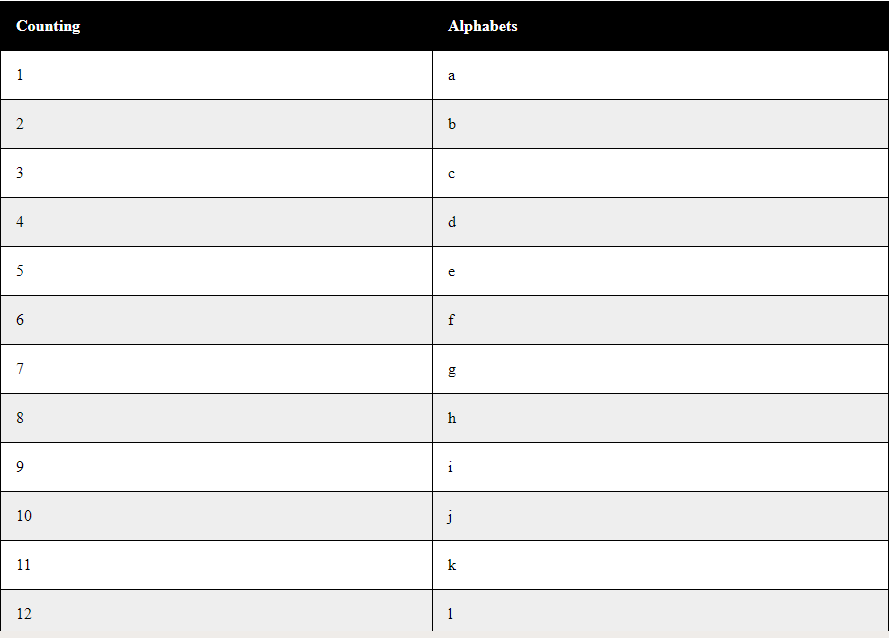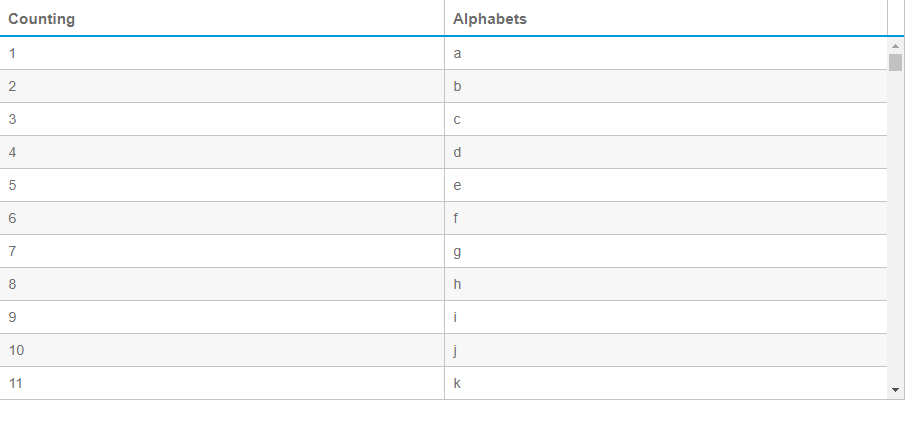# How to create data grid for millions of rows in JavaScript ?

In JavaScript, it is possible to show up to 105 using a simple loop. This also depends on the browser capability to run such a big loop with huge complexity. The page crashes when such a huge task is given to the browser. The code below is an example of how we can populate a table of 105 rows.

Example 1: This example will show how can we populate a table with 105 rows using a simple JavaScript code.

 ` ` `<``html``> ` ` `  `<``head``> ` `    ``<``style``> ` `        ``table { ` `            ``width: 100%; ` `        ``} ` `         `  `        ``table, ` `        ``th, ` `        ``td { ` `            ``border: 1px solid black; ` `            ``border-collapse: collapse; ` `        ``} ` `         `  `        ``th, ` `        ``td { ` `            ``padding: 15px; ` `            ``text-align: left; ` `        ``} ` `         `  `        ``table#t01 tr:nth-child(even) { ` `            ``background-color: #eee; ` `        ``} ` `         `  `        ``table#t01 tr:nth-child(odd) { ` `            ``background-color: #fff; ` `        ``} ` `         `  `        ``table#t01 th { ` `            ``background-color: black; ` `            ``color: white; ` `        ``} ` `    `` ` ` ` ` `  `<``body``> ` `    ``<``br``> ` ` `  `    ``<``table` `id``=``"t01"``> ` `        ``<``tr``> ` `            ``<``th``>Counting ` `            ``<``th``>Alphabets ` `        `` ` `        ``<``script``> ` `            ``var k = 97; ` `            ``for (var j = 1; j <= 100000; j++) { ` `                ``if (k == 123) ` `                    ``k = 97; ` `                ``var string = String.fromCharCode(k); ` `                ``document.write(" <``tr``><``td``>" + (j) + "<``td``>"  ` `                               ``+ string + ""); ` `                ``k++; ` `            ``} ` `        `` ` `    `` ` ` `  ` ` ` `  ` `

Output:Performance with 1 million rows
To perform such a heavy task we will be importing an API made by shield UI. It is a light weight API for loading big grids which actually loads the grids in real time i.e as we scroll down the table and thus decreasing the load on the browser at that instance of time.

Now let us perform the same operation for 1 million rows i.e 106 rows. The code below will load 1 million rows in real time manner.
Example:

 ` ` `<``html` `lang``=``"en"``> ` ` `  `<``head``> ` `    ``<``meta` `charset``=``"utf-8"` `/> ` `    ``<``title``>1 Million Rows Demo ` `    ``<``link` `id``=``"themecss"`  `          ``rel``=``"stylesheet"`  `          ``type``=``"text/css"`  `          ``href``= ` `"//www.shieldui.com/shared/components/latest/css/light/all.min.css"` `/> ` `    ``<``script` `type``=``"text/javascript"` `            ``src``= ` `"//www.shieldui.com/shared/components/latest/js/jquery-1.11.1.min.js"``> ` `  `` ` `    ``<``script` `type``=``"text/javascript"`  `            ``src``= ` `"//www.shieldui.com/shared/components/latest/js/shieldui-all.min.js"``> ` `  `` ` ` ` ` `  `<``body` `class``=``"theme-light"``> ` `    ``<``div` `id``=``"grid"` `style``=``"margin-bottom: -500px;"``> ` `    ``<``script` `type``=``"text/javascript"``> ` `        ``\$(function() { ` `            ``var grid = new shield.DataSource({ ` `                ``remote: { ` `                    ``operations: ["skip", "take"], ` `                    ``read: function(params, success, error) { ` `                        ``var skip = grid.skip || 0, ` `                            ``take = grid.take || 20, ` `                            ``data = [], ` `                            ``k = 96; ` ` `  `                        ``for (var i = skip; i < ``skip` `+ take; i++) { ` `                            ``k++; ` `                            ``var row = {}; ` `                            ``for (var ``j` `= ``1``; j < 3; j++) { ` `                                ``row["Counting"] = i + 1; ` `                                ``if (k == 123) ` `                                    ``k` `= ``97``; ` `                                ``var ``string` `= ``String``.fromCharCode(k); ` `                                ``row["Alphabets"] = string; ` `                            ``} ` `                            ``data.push(row); ` `                        ``} ` `                        ``success({ ` `                            ``data: data, ` `                            ``total: 1000000 ` `                        ``}); ` `                    ``} ` `                ``}, ` `                ``schema: { ` `                    ``data: "data", ` `                    ``total: "total" ` `                ``} ` `            ``}); ` `            ``\$("#grid").shieldGrid({ ` `                ``dataSource: grid, ` `                ``height: 400, ` `                ``scrolling: { ` `                    ``virtual: true ` `                ``}, ` `                ``columns: (function() { ` `                    ``var cols = []; ` ` `  `                    ``cols.push({ ` `                        ``field: "Counting", ` `                        ``width: 140 ` `                    ``}); ` ` `  `                    ``cols.push({ ` `                        ``field: "Alphabets", ` `                        ``width: 140 ` `                    ``}); ` `                    ``return cols; ` `                ``})() ` `            ``}); ` `        ``}); ` `    `` ` ` ` ` `  ` `

Output:My Personal Notes arrow_drop_upCheck out this Author's contributed articles.

If you like GeeksforGeeks and would like to contribute, you can also write an article using contribute.geeksforgeeks.org or mail your article to contribute@geeksforgeeks.org. See your article appearing on the GeeksforGeeks main page and help other Geeks.

Please Improve this article if you find anything incorrect by clicking on the "Improve Article" button below.